cancel
Showing results for
Did you mean:Frequent Visitor

## The XIRR function is not working

When trying to use the XIRR function, an error saying "The XIRR function couldn't find a solution" pops up. My data has both negative and positive values and also starts with a negative value and when I calculate it in Excel it works fine. The DAX query is as follows: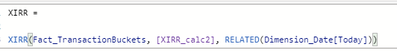1 ACCEPTED SOLUTIONSuper User

@Aymane Thanks for that 🙂

First of all, can you test this:

1. Create a simple table visual containing Dimension_Date[Today] and XIRR_Calc2.
2. Do you see the cashflow values that you are expecting to feed into the IRR calculation?

If so, you could rewrite the XIRR measure as:

``````XIRR =
XIRR (
VALUES ( Dimension_Date[Today] ), -- OR Dimension_Date
[XIRR_calc2],
Dimension_Date[Today]
)``````

If not, the logic may need to be rewritten in some way, either by modifying XIRR_Calc2 so that it returns the required cashflow when grouped by date, or adding some logic to the XIRR measure.

There are a couple of points to consider here:

1. Iterating over a fact table with XIRR will likely give an unexpected result, due to the risk of duplicated rows. For this reason (and performance reasons) it's best to iterate over a dimension table (or dimension column).
2. We need to ensure that XIRR_Calc2 returns the expected values for each row of the table provided in the first argument.  Given that there is some complexity, with vMin and vMax being evaluated within the measure, there might be some tweaks required.

To explain further:

With your current XIRR measure, the XIRR_Calc2 measure is being evaluated in the row context of every row of Fact_TransactionBuckets. Due to context transition (since a measure is being evaluated), each row of Fact_TransactionBuckets is converted into an equivalent filter context, and if there happen to be any duplicated rows, things could go awry (good article on context transition here).

Regards,

Owen

Owen Auger
Blog
12 REPLIES 12Frequent Visitor

Thank you for the response @OwenAuger,

The relationship between the Dimension_Date and Fact_TransactionBuckets is indeed 1:many relationship.

The [XIRR_calc2] is as follows:This code is working and giving the correct values for the cashflows.
As for the Fact_TransactionBuckets, it includes an asofdatekey that connects it to the Dimension_Date, and values that are used to calculate [XIRR_calc2] that you can see in the screenshot.Super User

@Aymane Thanks for that 🙂

First of all, can you test this:

1. Create a simple table visual containing Dimension_Date[Today] and XIRR_Calc2.
2. Do you see the cashflow values that you are expecting to feed into the IRR calculation?

If so, you could rewrite the XIRR measure as:

``````XIRR =
XIRR (
VALUES ( Dimension_Date[Today] ), -- OR Dimension_Date
[XIRR_calc2],
Dimension_Date[Today]
)``````

If not, the logic may need to be rewritten in some way, either by modifying XIRR_Calc2 so that it returns the required cashflow when grouped by date, or adding some logic to the XIRR measure.

There are a couple of points to consider here:

1. Iterating over a fact table with XIRR will likely give an unexpected result, due to the risk of duplicated rows. For this reason (and performance reasons) it's best to iterate over a dimension table (or dimension column).
2. We need to ensure that XIRR_Calc2 returns the expected values for each row of the table provided in the first argument.  Given that there is some complexity, with vMin and vMax being evaluated within the measure, there might be some tweaks required.

To explain further:

With your current XIRR measure, the XIRR_Calc2 measure is being evaluated in the row context of every row of Fact_TransactionBuckets. Due to context transition (since a measure is being evaluated), each row of Fact_TransactionBuckets is converted into an equivalent filter context, and if there happen to be any duplicated rows, things could go awry (good article on context transition here).

Regards,

Owen

Owen Auger
BlogFrequent Visitor

Hi,

I am facing the similar issue as explained by original author. I tried your solution but it is not working.

Formula I used:

XIRR(VALUES('Year'[Year_Date]), [Measure 1]-SUM('Table'[Value]),'Year'[Year_Date],10)
where, 'Year' is a dimension table with 5 consecutive years in date format (eg: 1/1/2020)
[Measure 1] - is a measure being calculated from multiple tables
SUM('Table'[Value]) - yearly flat value coming from a single fact table

I tried making a simple table with years and required values, it works fine. XIRR formula does not work and shows an error 'The XIRR function couldn't find a solution.'

Thanks!Super User

Two things I can see that may be the source of the error:

1. SUM(...) should be wrapped in CALCULATE if it should be evaluated with a particular Year_Date filter applied, which would be the case if 'Table' has a relationship with the 'Year' table.
2. The guess parameter of 10 seems high (=1,000%). Does it work better with a lower guess, e.g. 0.1 (=10%).

With these changes, the measure would be something like:

``````XIRR Measure =
XIRR (
VALUES ( 'Year'[Year_Date] ),
[Measure 1] - CALCULATE ( SUM ( 'Table'[Value] ) ),
'Year'[Year_Date],
0.1
)``````

If you are still getting errors, could you possibly post some sample data or a sanitised PBIX?

Regards,

Owen

Owen Auger
BlogFrequent Visitor

Thank you so much!!! This worked! Adding 'Calculate' function worked with the formula.

I did have relationship build between the tables and I had tried the formula without the guess figure, but it wasn't working. Calculate formula was all I was missing!Frequent Visitor

Hi @OwenAuger , Thank you again for your solution.

I have another issue regarding the same problem, after calculating the XIRR, I need to create a visualization table where XIRR is filtered by another column(AssetClass) in another table (Dimension_Asset).

Usually, all the calculations I've made have been done on the Fact_TransactionBuckets which has an AssetKey that connects it with the Dimension_Asset, however, since the Dimension_date Table (Where the XIRR was calculated) doesn't have a direct relationship with Dimension Asset, the XIRR can not be filtered by the column AssetClass.

Can you please suggest a solution that doesn't involve adding or changing anything in the model?Super User

You're welcome @Aymane  🙂

If your data model has been set up the way you've described, with Fact_TransactionBuckets related to both Dimension_Asset and Dimension_Date, then filters on either of those dimension tables should filter Fact_TransactionBuckets and the XIRR measure should respond accordingly.

To help me understand the issue, could you post a sanitised version of the PBIX with dummy data?

I wasn's sure what you meant when you say the calculation was does "in" the Dimension_date or Fact_TransactionBuckets tables? The measure does reference those tables, but it should return the same result regardless of its home table.

Also just want to check I've understood you correctly and you have created a measure rather than a calculated column.

Regards,

Owen

Owen Auger
BlogFrequent Visitor

Hi @OwenAuger ,

I have created a dummy data model as follows: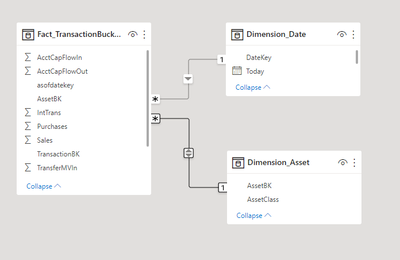For the XIRR, I created a measure in the Fact_TransactionBuckets; when I put the measure into a Table, it works fine, however, when I add AssetClass to it, the following error message is prompted: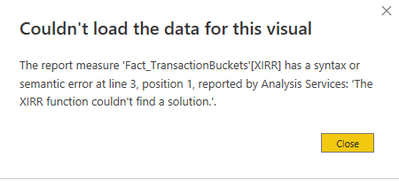The same doesn't happen when I use other measures added to Fact_TransactionBuckets instead of XIRR, example:Super User

Thanks @Aymane

Having re-looked at the XIRR_calc2 measure, as it in part references min/max dates, from Dimension_Date, one solution might be to derive a date filter from Fact_TransactionBuckets and apply that to the overall calculation.

Something like:

``````XIRR =
CALCULATE (
XIRR (
VALUES ( Dimension_Date[Today] ),
-- OR Dimension_Date
[XIRR_calc2],
Dimension_Date[Today]
),
SUMMARIZE (
Fact_TransactionBuckets,
Dimension_Date[Today]
)
)``````

If you could share a PBIX with dummy data and expected result for a given Asset filter, I may be able to give a more watertight answer.

Regards,

Owen

Owen Auger
BlogFrequent Visitor

Hi @OwenAuger ,

I have found a solution to this issue by using calculating XIRR for each asset class by using switch(TRUE() and filtering, however some of the calculations that I have got are incorrect.

The reason for that is that in [XIRR_calc2] we are using vMin when we should be using the first non blank value and not the first value in calculation.

I have created a measure that returns the first non blank value date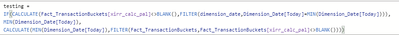The [xirr_calc_pal] is just a copy of [XIRR_calc2].

This measure works fine and gives the following results: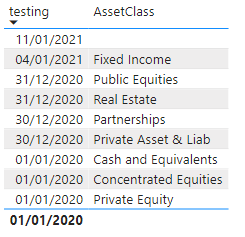, but I don't know how to use this measure instead of [vMin] in the [XIRR_calc2].Frequent Visitor

Thank you @OwenAuger , it's working great.Super User

Hi @Aymane

What is the content & granularity of the Fact_TransactionBuckets table (maybe post a sample), and what is the definition of [XIRR_calc2]?

Also presumably there's a typical 1:many relationship between Dimension_Date and Fact_TransactionBuckets? (Otherwise RELATED would have thrown an error.)

I usually ensure that the first argument of XIRR contains one row per distinct date for performance reasons, though this isn't strictly necessary.

Regards,

Owen

Owen Auger
BlogAnnouncements#### Exclusive opportunity for Women!

Join us for a free, hands-on Microsoft workshop led by women trainers for women where you will learn how to build a Dashboard in a Day!#### Power Platform Conference-Power BI and Fabric Sessions

Join us Oct 1 - 6 in Las Vegas for the Microsoft Power Platform Conference.Top Solution Authors
Top Kudoed Authors
Users online (3,849)